# FastDVDNet Trained onDAVIS Data

Remove noise from a video

Released in 2019, this network achieves state-of-the-art results on video denoising. FastDVDNet outruns its competitors by faster inference time and the ability to handle a wide range of noise levels within a single model.

Number of layers: 237 | Parameter count: 2,488,008 | Trained size: 11 MB |

## Performance

• The model achieves 31.86 PSNR and 28.89 ST-RRED on the Set8 and DAVIS testset.

## Examples

Get the pre-trained net:

 In:=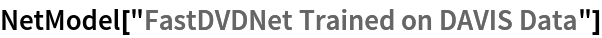Out=### Basic usage

Get a noisy video:

 In:=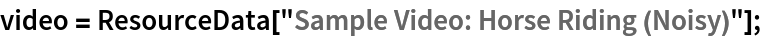Define a noise level for a video:

 In:=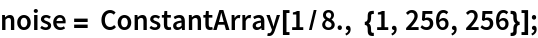Denoise a video:

 In:=Out=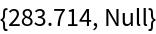Visualize the five frames of a noisy and denoised video:

 In:=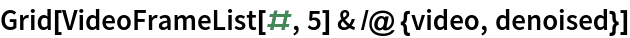Out=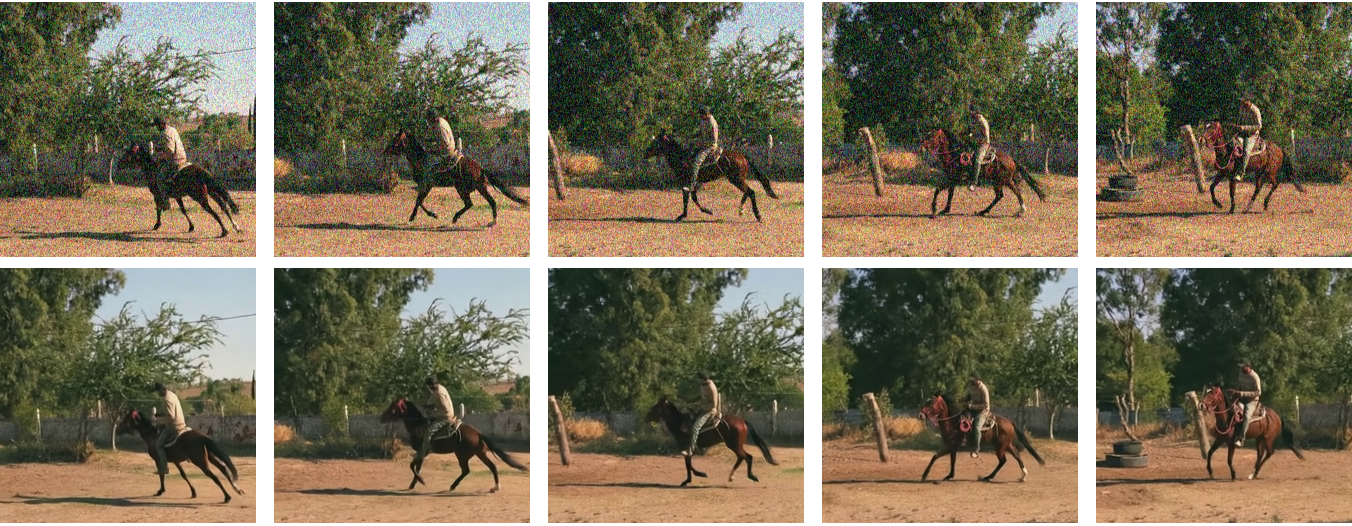Automatic frame resizing can be avoided by replacing the NetEncoder. First get the net:

 In:=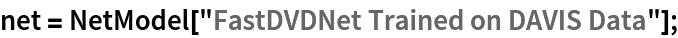Get a noisy video:

 In:=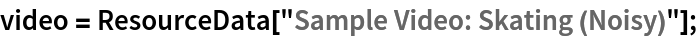Create a new NetEncoder with the desired dimensions (to get a resizable net, the spatial dimensions should be divisible by 4):

 In:=Out=Attach the NetEncoder, define a noise level and run the net:

 In:=Visualize the three frames of a noisy and denoised video:

 In:=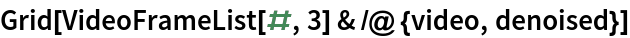Out=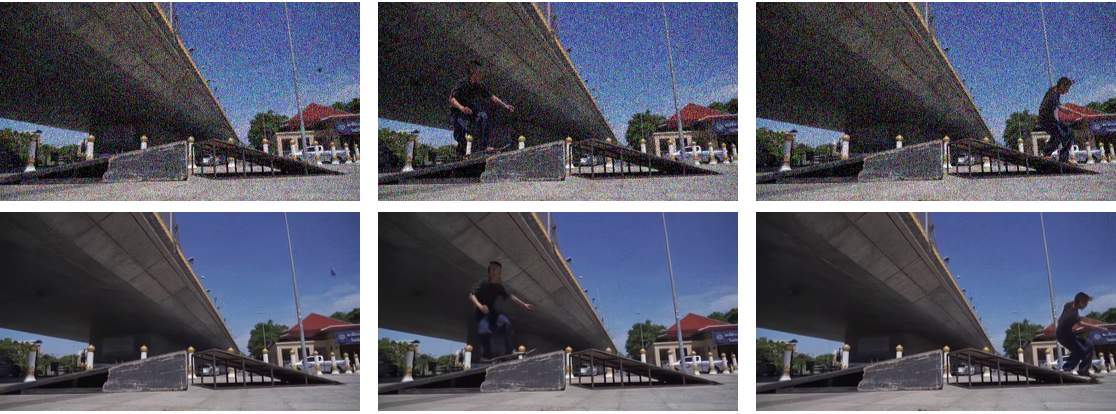### FastDVDNet architecture

The video encoder takes the first five frames of the input video and encodes it into a vector of size (5,3,256,256):

 In:=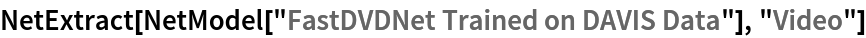Out=Note that the first three blocks are shared. (Framei, Framei+1, Framei+2, Noise) are given as inputs to blocki to obtain an intermediate denoised frame to obtain an intermediate denoised output frame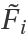. Finally, (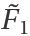,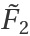,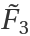, Noise) are given as inputs to the last block to get the final denoised output frame:

 In:=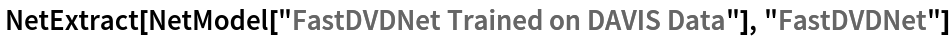Out=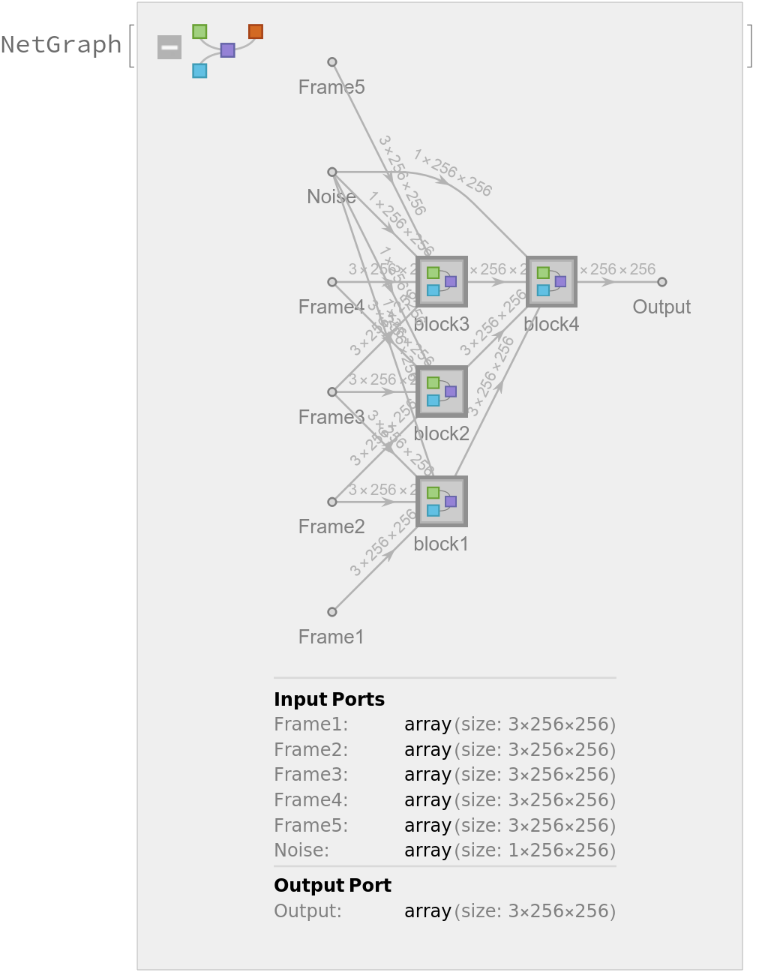Extract the first and the last blocks of the net:

 In:=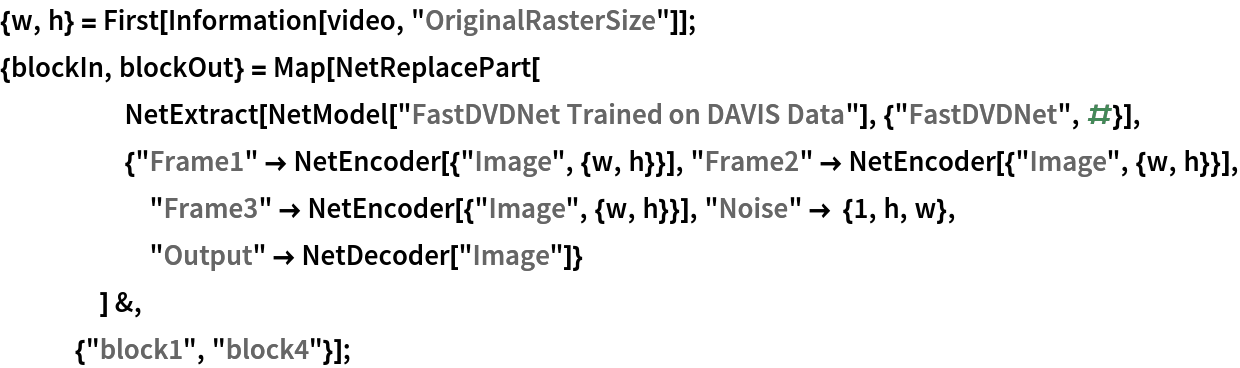All the blocks share a U-Net type architecture. Explore the input block:

 In:=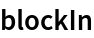Out=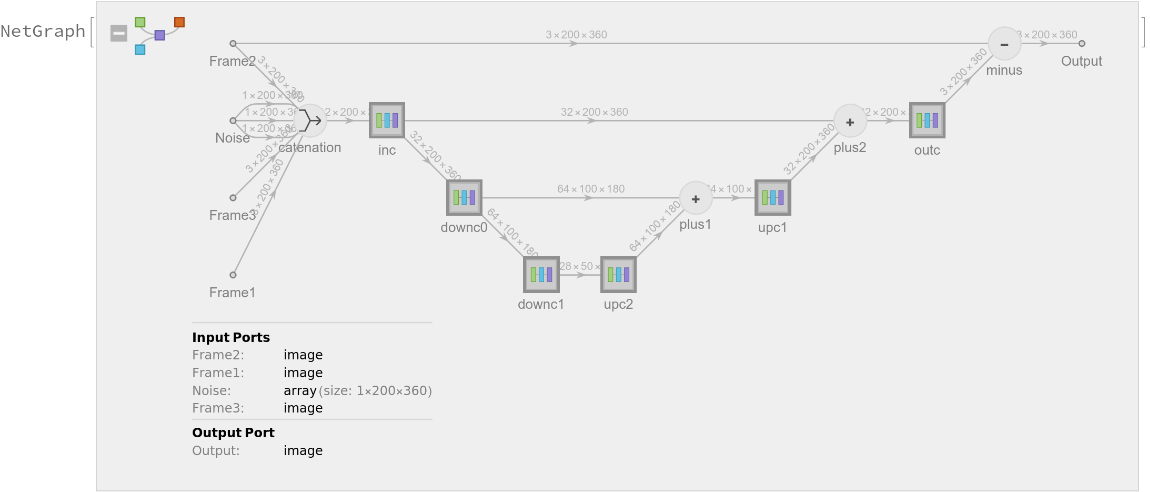Get a noisy video and define a noise level for a video:

 In:=Simply mapping the net to a list of frames can be inefficient due some blocks processing the same frame multiple times. To avoid double counting, split the inference into two steps:

 In:=Get the final denoised video:

 In:=Visualize the three frames of a noisy video and the intermediate and final result:

 In:=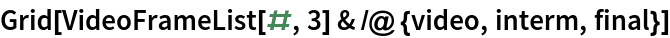Out=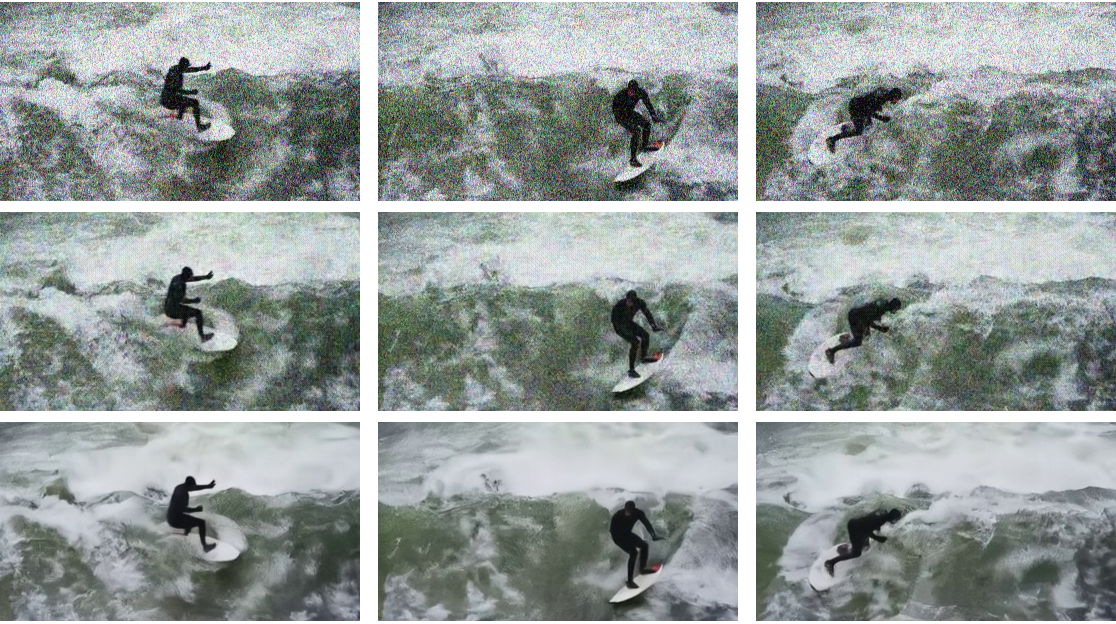### Memory-efficient evaluation

Define a custom videoDenoise function for memory-efficient processing of video with Gaussian or Poisson noise:

 In:=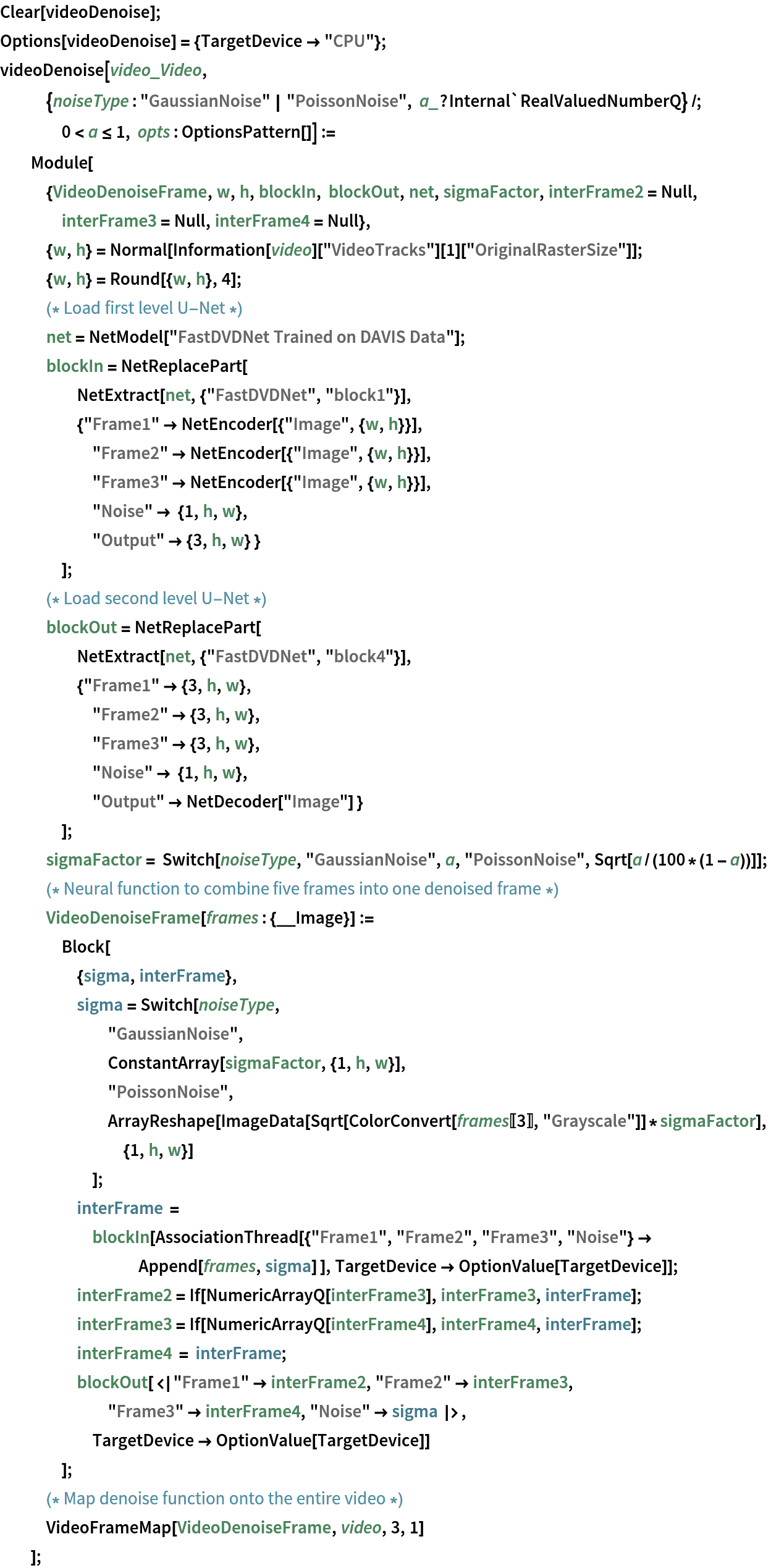Get a noisy video:

 In:=Get a final denoised video assuming a Gaussian distribution on the input noise:

 In:=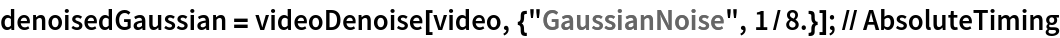Out=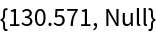Get a final denoised video assuming a Poisson distribution on the input noise:

 In:=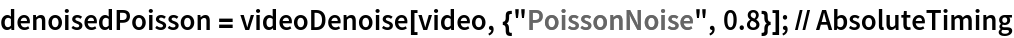Out=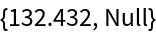Visualize the four frames of a noisy video and the denoised results assuming a Gaussian and Poisson distribution on the input noise:

 In:=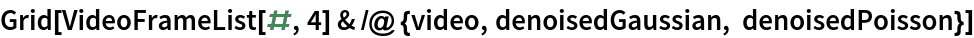Out=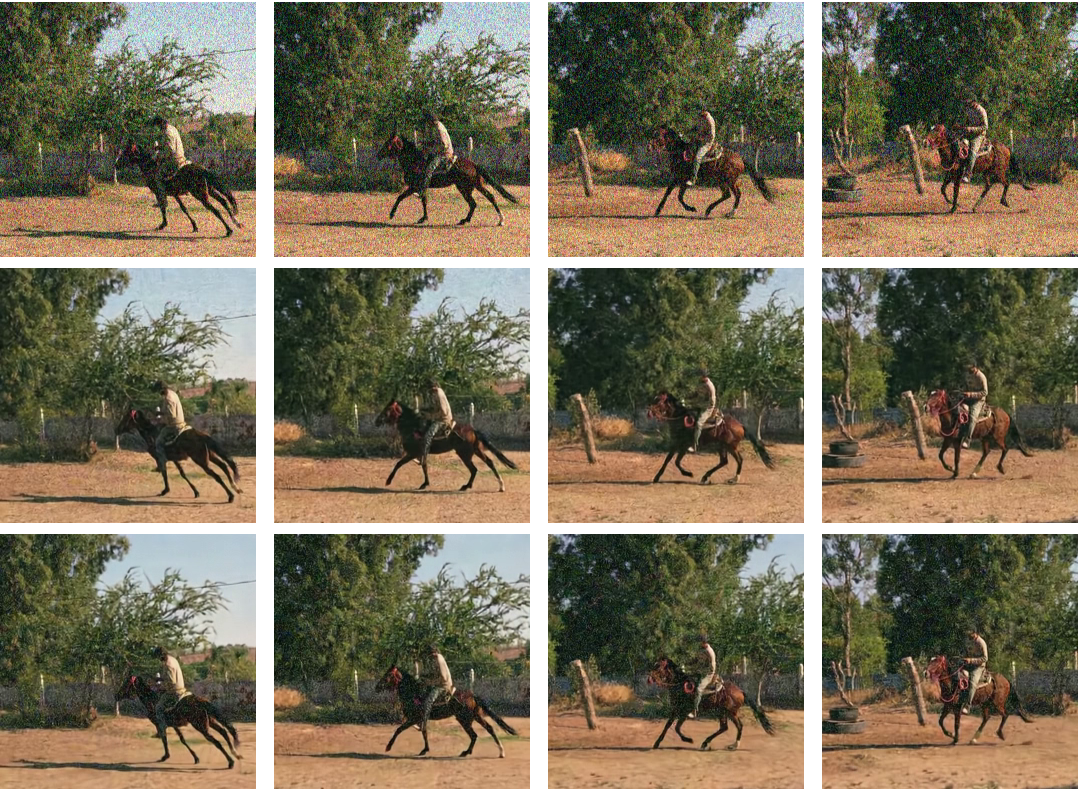### Net information

Inspect the number of parameters of all arrays in the net:

 In:=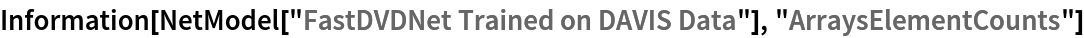Out=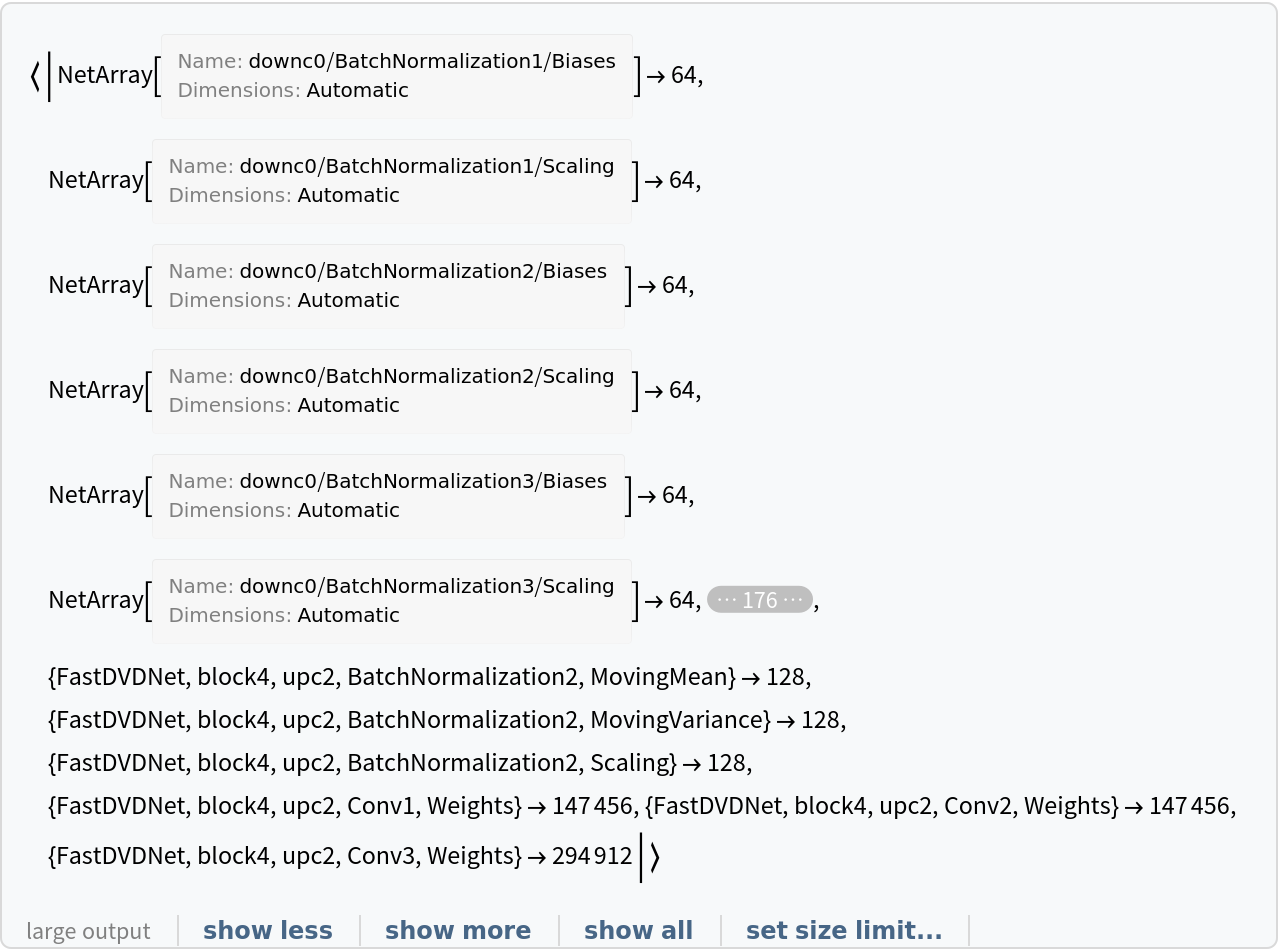Obtain the total number of parameters:

 In:=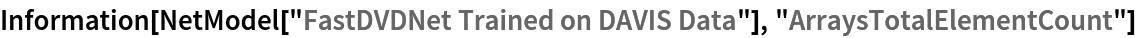Out=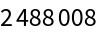Obtain the layer type counts:

 In:=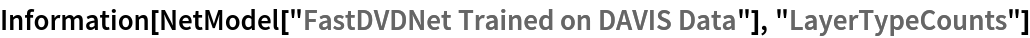Out=Display the summary graphic:

 In:=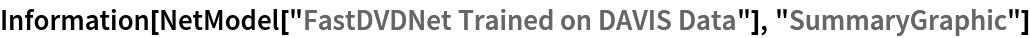Out=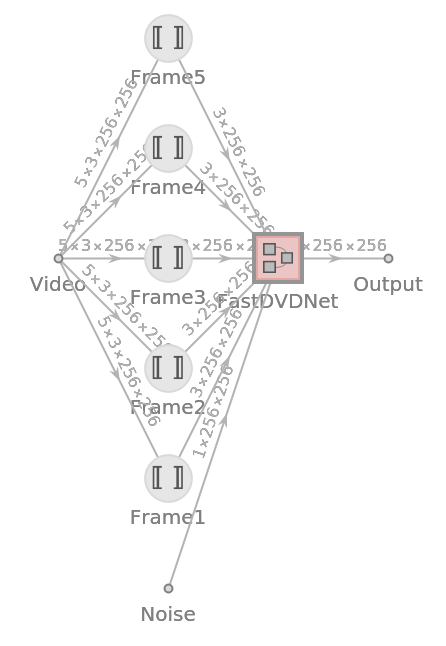### Export to ONNX

Export the net to the ONNX format:

 In:=Out=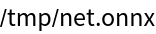Get the size of the ONNX file:

 In:=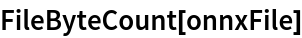Out=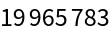The byte count of the resource object is smaller because shared arrays are currently being duplicated when exporting to ONNX:

 In:=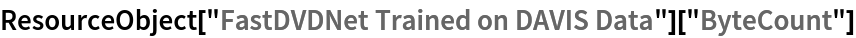Out=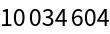Check some metadata of the ONNX model:

 In:=Out=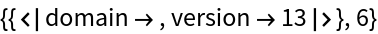Import the model back into the Wolfram Language. However, the NetEncoder and NetDecoder will be absent because they are not supported by ONNX:

 In:=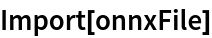Out=## Reference

• M. Tassano, J. Delon, T. Veit, "FastDVDnet: Towards Real-Time Deep Video Denoising Without Flow
Estimation," arXiv:1907.01361 (2019)
• Available from:
• Rights: Copying and distribution of this file, with or without modification,
are permitted in any medium without royalty provided the copyright
notice and this notice are preserved. This file is offered as-is,
without any warranty.
• Author: Matias Tassano, mtassano at gopro dot com